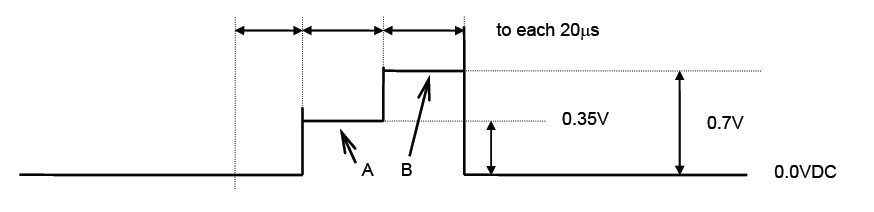### Product Summary

The LA76810A is a SANYO semiconductor.

### Parametrics

LA76810A characteristics: (1)On the conditions of V8 = 5V, V3 = 2.5V, IF supply current I8: typ=65.0mA; (2)On the conditions of I18 = 19mA, RGB supply voltage V18: typ=8.0V; (3)On the conditions of I25 = 27mA, Horizontal supply voltage V25: typ=5.0V; (4)On the conditions of I31 = 5V, CCD supply current I31: typ=5.6mA; (5)On the conditions of I43 = 5V, Video supply current I43: typ=124.0mA; (6)Voltage gain R GV_R: min=-2dB, typ=0, max=2dB; (7)Voltage gain B GV_B: min=-2dB, typ=0, max=2dB; (8)Difference of voltage gain DGV: min=0, typ=0.1dB, max=0.3dB; (9)Delay time Td: typ=63.8μs.

### DiagramsImage Part No Mfg DescriptionPricing
(USD)
QuantityLA76810AOtherData SheetNegotiable
Image Part No Mfg DescriptionPricing
(USD)
QuantityLA7605AMOtherData SheetNegotiableLA7605MOtherData SheetNegotiableLA76070OtherData SheetNegotiableLA76075OtherData SheetNegotiableLA7615OtherData SheetNegotiableLA7625OtherData SheetNegotiable